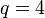# Element structure of unitriangular matrix group:UT(3,4)

## Contents

View element structure of particular groups | View other specific information about unitriangular matrix group:UT(3,4)

## Summary

Item Value
number of conjugacy classes 19
As$UT(3,q), q = 4$:$q^2 + q - 1 = 4^2 + 4 - 1 = 19$
order 64
As$UT(n,q), q = 4, n = 3$:$q^{n(n-1)/2} = 4^{3(2)/2} = 4^3 = 64$
exponent 4
As$UT(3,q), q = 4$ is a power of 2: 4
conjugacy class size statistics size 1 (4 classes), size 4 (15 classes)
order statistics order 1 (1 element), order 2 (27 elements), order 4 (36 elements)

## Conjugacy class structure

### Interpretation as unitriangular matrix group of degree three

Compare with element structure of unitriangular matrix group of degree three over a finite field
Nature of conjugacy class Jordan block size decomposition Minimal polynomial Size of conjugacy class (generic$q$) Size of conjugacy class ($q = 4$) Number of such conjugacy classes (generic$q$) Number of such conjugacy classes ($q = 4$) Total number of elements (generic$q$) Total number of elements ($q = 4$) Order of elements in each such conjugacy class (generic$q$) Order of elements in each such conjugacy class ($q = 4$, so$p = 2$) Type of matrix
identity element 1 + 1 + 1 + 1$x - 1$ 1 1 1 1 1 1 1 1$a_{12} = a_{13} = a_{23} = 0$
non-identity element, but central (has Jordan blocks of size one and two respectively) 2 + 1$(x - 1)^2$ 1 1$q - 1$ 3$q - 1$ 3$p$ 2$a_{12} = a_{23} = 0$,$a_{13} \ne 0$
non-central, has Jordan blocks of size one and two respectively 2 + 1$(x - 1)^2$$q$ 4$2(q - 1)$ 6$2q(q - 1)$ 24$p$ 2$a_{12}a_{23} = 0$, but not both$a_{12}$ and$a_{23}$ are zero
non-central, has Jordan block of size three 3$(x - 1)^3$$q$ 4$(q - 1)^2$ 9$q(q - 1)^2$ 36$p$ if$p$ odd
4 if$p = 2$
4 both$a_{12}$ and$a_{23}$ are nonzero
Total (--) -- -- -- --$q^2 + q - 1$ 19$q^3$ 64 -- -- --